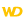HelpWLanguageWLanguage functionsControls, pages and windowsDrawing functionsPrefix syntaxDrawing functions on picLayer variablesDrawChordPresentationExampleLimitationsCoordinatesSolid figureDrawing in PHPDrawing in Browser codeRelated examplesSee alsoAlphaBlendBackgroundBlurChangeModeDrawArcDrawChordDrawCircleDrawLineDrawPointDrawPolygonDrawPolylineDrawRectangleDrawRectangleGradientDrawRoundedRectangleDrawSliceDrawTextDrawTextRTFEndDrawingFillFontHorizontalSymmetryInvertColorPenPixelColorPixelOpacityResizeRotationStartDrawingVerticalSymmetry
• Limitations
• Coordinates
• Solid figure
• Drawing in PHP
• Drawing in Browser code
WINDEVWEBDEVWINDEV MobileOthers<picLayer variable>.DrawChord (Function)
In french: <Variable picCalque>.DessineCorde
Draws the chord of a circle (intersection between an ellipse and a straight line) in a variable of type picLayer.
Example
MyImage is WDPic = "Test.gif"
MyLayer is picLayer

// In a layer, draw the chord of a circle whose
// background is light yellow and whose border is light blue
MyLayer.DrawChord(10, 10, 50, 50, 20, 20, 40, 50, LightYellow, LightBlue)

IMG_MyDrawing = MyImage
Syntax
<picLayer image>.DrawChord(<X1> , <Y1> , <X2> , <Y2> , <X3> , <Y3> , <X4> , <Y4> [, <Background color> [, <Line color>]])
<picLayer image>: picLayer variable
Name of the picLayer variable to be used.
<X1>: Integer
X-coordinate of the upper-left corner of the rectangle containing the circle. These coordinates are expressed in pixels.
<Y1>: Integer
Y-coordinate of the upper-left corner of the rectangle containing the circle. These coordinates are expressed in pixels.
<X2>: Integer
X-coordinate of the lower-right corner of the rectangle containing the circle. These coordinates are expressed in pixels.
<Y2>: Integer
Y-coordinate of the lower-right corner of the rectangle containing the circle. These coordinates are expressed in pixels.
<X3>: Integer
X-coordinate of the start point of the chord. These coordinates are expressed in pixels.
<Y3>: Integer
Y-coordinate of the start point of the chord. These coordinates are expressed in pixels.
<X4>: Integer
X-coordinate of the end point of the chord. These coordinates are expressed in pixels.
<Y4>: Integer
Y-coordinate of the end point of the chord. These coordinates are expressed in pixels.
<Background color>: Integer or constant (optional)
Background color of the chord. This color can correspond to:
• an RGB color (returned by RGB),
• an HSL color (returned by HSL),
• a WLanguage preset color,
•a variable of type Color.
If this parameter is not specified, the background color:
<Line color>: Integer or constant (optional)
Line color for the circle chord. This color can correspond to:
• an RGB color (returned by RGB),
• an HSL color (returned by HSL),
• a WLanguage preset color,
•a variable of type Color.
If this parameter is not specified, the line color:
• corresponds to the color specified in the last call to <Image>.Pen,
• is the same as the background color if <Image>.Pen has not been used.
Remarks

Limitations

• <picLayer variable>.DrawChord is not available in anti-aliasing mode (for more details, see the help about <picLayer variable>.ChangeMode).
• <picLayer variable>.DrawChord is not available for a drawing with management of alpha channel (for more details, see the help about <picLayer variable>.StartDrawing). This function has no effect.

Coordinates

If the start and end points are not located on the outline of the circle (or on the outline of the ellipse), the point taken into account is the intersection between the circle and the line that joins the specified point and the center of the rectangle (which means the center of the circle).The coordinates are specified with respect to the upper-left corner of the Image control (coordinates set to (0,0)).

Solid figure

<picLayer variable>.DrawChord can only be used to draw a chord: outline only or chord area. To draw a solid figure (portion defined by the center of the circle and the two points on the circle), use <picLayer variable>.DrawSlice.
Related Examples:Unit examples (WINDEV): The drawing functions [ + ] Using the main drawing functions of WINDEV to:- Initialize an Image control for drawing- Draw simple shapes- Write a text into a drawing- Change the color in a drawing
Business / UI classification: Neutral code
Component: wd280pnt.dll
Minimum version required
• Version 25